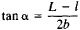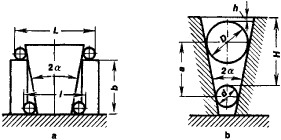Angle-Measuring Instrument

Angle-Measuring Instrument

in machine building, any one of a group of devices used to measure angles.

Angle-measuring instruments may be classified into four types according to the measuring method used. The first group includes devices that compare the angle to be measured against a fixed gage, such as an angle gage (for angles 1’ to 180°), try square (usually for a 90° angle), tapered plug gage, or template. Such instruments characteristically have a fixed angle or angles. They may be laid directly on the surfaces whose angles are being measured, in which case the contact gap can be measured visually or by means of a telltale dye, or they may be used to zero the readout of some monitoring device.

Instruments in the second group measure an unknown angle by comparing it with an angle preset on the measuring device; examples are tangent and sine bars, which are adjusted for the tangent or sine of the measured angle or complementary angle. A readout is used to compare the measured angle with the preset angle, from which the deviation is determined.

Instruments of the third type compare the angle to be measured with an angular scale; these include bevel protractors, optical goniometers, index heads and index tables, theodolites, quadrants, and goniometers. The readout scales of such instruments usually have scale divisions of 1” to 2’ in the range from 0° to 180° or 360°. The instruments may be mounted on the part being measured, or the part may be mounted on the instrument. Special devices make it possible to align the angle being measured in a position where it can be compared with an angle preset on the instrument’s scale.

The fourth group consists of instruments that measure one side of a right triangle while one other side is kept constant; trigonometric sine and tangent functions are then used to find the value of the unknown angle. This is called indirect measurement. Such measurements are made with the aid of measuring microscopes, coordinate-measuring machines, and other special devices. Conical holes, frustums, and cones may be measured with balls and rollers (Figure 1). For frustums, the dimension L across the rollers at the greater base is first determined with a measuring device. The dimension l at the lesser base is then measured, and the height b is determined with the aid of gage blocks (Figure 1, a). From the equationthe angle α is determined and compared with the specified angle. In order to determine the angle of a conical hole (Figure 1, b), the lengths h and H are measured to the tops of the larger and the smaller balls, respectively, which have the known dimensions D and d. From the measured distance a between the balls, the sine of the angle is found as sin α = d/(2a - D + d).Figure 1. Measurement of angles with rollers and balls, (a) Measurement with rollers: (L) distance across the rollers at the greater base of the frustum, (I) distance across the rollers at the lesser base, (b) distance between measured cross sections; (b) measurement with balls: (h) distance from the greater base of the conical hole to the upper surface of the larger ball, (H) distance from the greater base to the upper surface of the smaller ball, (D) diameter of (he larger ball, (d) diameter of the smaller ball, (a) distance between the centers of the balls

Certain angle-measuring instruments currently in the development stage compare the angle to be measured with a graduated, angular scale. They may use a system of induction meters built into levels or index heads or index tables, as well as magnetic and grating scales. The use of such instruments facilitates the automation of measurement processes and the recording of results. Indirect measurement is the preferred method for automatically controlled measurements.

REFERENCES

Eidinov, V. Ia. Izmerenie uglov v mashinostroenii. Moscow, 1963.
Opticheskie pribory dlia izmereniia lineinykh i uglovykh velichin v mashinostroenii. Moscow, 1964.
Optiko-mekhanicheskiepribory. Moscow, 1965.

N. N. MARKOV

References in periodicals archive ?
The surface wettability of PCL/HA composite was investigated by measuring the static contact angle after dispensing a deionized water drop of 2 [micro]L on the solid flat surface for 30 sec using a surface contact angle-measuring instrument (OCA 30, Dataphysics Company, Germany).
The water absorption process was also recorded after releasing a deionized water droplet of 2 [micro]L onto the surface of scaffold by using the contact angle-measuring instrument (OCA 30, Dataphysics Company).
was constantly returning to the task of improving angle-measuring instruments.

Site: Follow: Share:
Open / Close